• +91 9971497814
• info@interviewmaterial.com

# Chapter 4 Linear Equations in Two Variables Ex-4.4 Interview Questions Answers

### Related Subjects

Question 1 :

Give the geometric representation of y = 3 as anequation

(I) in one variable

(II) in two variables

In one variable, y = 3 represents a point as shown infollowing figure.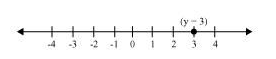In two variables, y = 3 represents a straight linepassing through point (0, 3) and parallel to x-axis. It is acollection of all points of the plane, having their y-coordinate as3.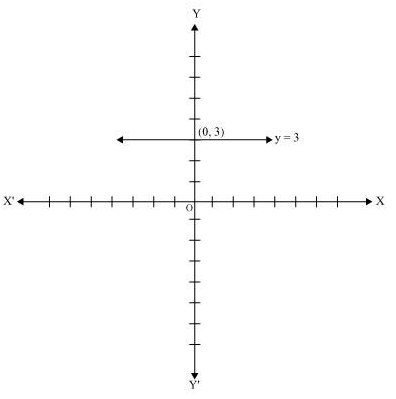Question 2 :

Give the geometric representations of 2x + 9 = 0 as anequation

(1) in one variable

(2) in two variables

Answer 2 : (1)In one variable, 2x + 9 = 0 represents a point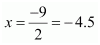as shown in the following figure.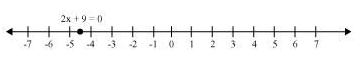(2) In two variables, 2x + 9 = 0 represents a straight linepassing through point
(−4.5, 0) and parallel to y-axis. It is a collection of all pointsof the plane, having their x-coordinate as 4.5.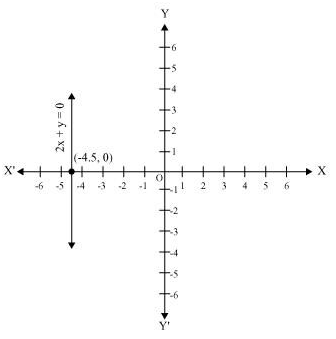Todays Deals### Chapter 4 Linear Equations in Two Variables Ex-4.4 Contributorskrishan

Name:
Email:

# Latest News# 9000 interview questions in different categories